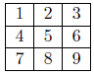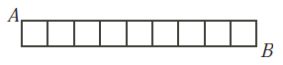# 2007COMC加拿大数学公开赛真题免费下载

### 真题与答案下载### 2007 COMC真题免费下载

Part A共8题，每题5分

Part B共4题，每题10分

#### 部分真题预览：

Part A :

4)In June, the ratio of boys to girls in a school was 3 : 2. In September, there were 80 fewer boys and 20 fewer girls in the school and the ratio of boys to girls was 7 : 5. What was the total number of students at the school in June?

5)The numbers 1, 2, 3, . . . , 9 are placed in a square array. The sum of the three rows, the sum of the three columns, and the sum of the two diagonals are added together to form a “grand sum”, S. For example, if the numbers are placed as shown, the grand sum is S = row sums + column sums + diagonal sums = 45 + 45 + 30 = 120 .What is the maximum possible value of the grand sum S?8)In the diagram, there are 28 line segments of length 1 arranged as shown to form 9 squares. There are various routes from A to B travelling along the segments so that no segment is travelled more than once. Of these possible routes, determine

• the length of route that occurs the most often, and
• the number of different routes of this length.Part B :

1)An arithmetic sequence a, a + d, a + 2d, . . . is a sequence in which successive terms have a common difference d. For example, 2, 5, 8, . . . is an arithmetic sequence with common difference d = 3 because 5 − 2 = 8 − 5 = 3.

1. If x − 1, 2x + 2 and 7x + 1 are the first three terms of an arithmetic sequence, determine the value of x.
2. For the value of x from (a), what is the middle term of the arithmetic sequence x − 1, 2x + 2, 7x + 1, . . . , 72?
A geometric sequence a, ar, ar2, . . . is a sequence in which successive terms have a common ratio r. For example, the sequence 2, 10, 50, . . . is a geometric sequence with common ratio r = 5 because10/2=50/10= 5.
3. If y − 1, 2y + 2 and 7y + 1 are the first three terms of a geometric sequence, determine all possible values of y.
4. For each of the values of y from (c), determine the 6th term of the geometric sequence y − 1, 2y + 2, 7y + 1, . . . .

### 2007 COMC加拿大数学奥赛完整版答案免费下载